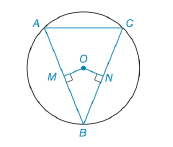Chapter 6.3, Problem 28E### Elementary Geometry for College St...

6th Edition
Daniel C. Alexander + 1 other
ISBN: 9781285195698

#### Solutions

Chapter
Section### Elementary Geometry for College St...

6th Edition
Daniel C. Alexander + 1 other
ISBN: 9781285195698
Textbook Problem
1 views

# In Exercises 27 to 30, provide a paragraph proof.Given: ⊙ O   with O M ¯ ⊥ A B ¯     a n d     O N ¯ ⊥ B C ¯ O M ¯ ≅ O N ¯ Secant A C   ¯   t o     ⊙ O Secant A E   ¯   t o     ⊙ Q Prove: Δ A B C is isosceles.To determine

To find:

To prove ΔABC is isosceles.

Explanation

Given that, O with OM¯AB¯andON¯BC¯ and OM¯ON¯.

To prove ΔABC is isosceles that is an isosceles triangle is a triangle that has two sides of equal length.

The diagrammatic representation is given below,

First compare the ΔONB,ΔOMB

But OB=OB. Since its common for both triangles and identity.

Given that OM¯AB¯andON¯BC¯

Therefore, OMB=ONB=90

(SAS) Definition: Triangles are congruent if any pair of corresponding sides and their included angles are equal in both triangles.

So by using side angle side property ΔOMBΔONB.

Also using CPCTC (corresponding parts of congruent triangles are congruent) to get the following,

MB¯=NB¯...(1)

Theorem 6.3.1:

If a line is drawn through the center of a circle perpendicular to a chord, then it bisects the chord and its arc.

That is for circle O given OM¯AB¯, therefore AM¯=MB¯...(2)

### Still sussing out bartleby?

Check out a sample textbook solution.

See a sample solution

#### The Solution to Your Study Problems

Bartleby provides explanations to thousands of textbook problems written by our experts, many with advanced degrees!

Get Started

#### Find more solutions based on key concepts